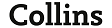# symmetric definición, symmetric significado | diccionario de inglés definición

Buscar también en: Web Noticias Enciclopedia Imágenes## symmetric

adj
1      (Logic, maths)   (of a relation) holding between a pair of arguments x and y when and only when it holds between y and x, as ... is a sibling of ... but not ... is a brother of ...
Compare       asymmetric       5       antisymmetric       nonsymmetric
2       another word for       symmetrical       5

symmetric matrix
n     (Maths)   a square matrix that is equal to its transpose, being symmetrical about its main diagonal. A skew symmetric matrix is equal to the negation of its transpose
Compare       orthogonal matrixsymmetrical
adj
1    possessing or displaying symmetry
Compare       asymmetric
2      (Maths)
a    (of two points) capable of being joined by a line that is bisected by a given point or bisected perpendicularly by a given line or plane
the points (x, y) and (--x, --y) are symmetrical about the origin
b    (of a configuration) having pairs of points that are symmetrical about a given point, line, or plane
a circle is symmetrical about a diameter
c    (of an equation or function of two or more variables) remaining unchanged in form after an interchange of two variables
x + y = z is a symmetrical equation
3      (Chem)   (of a compound) having a molecular structure in which substituents are symmetrical about the molecule
4      (Botany)      another word for       actinomorphic
5      (Also)    symmetric          (of a disease, infection, etc.) affecting both sides of the body or corresponding parts, such as both legs
symmetrically      adv
symmetricalness      n

symmetric
adj
1      (Logic, maths)   (of a relation) holding between a pair of arguments x and y when and only when it holds between y and x, as ... is a sibling of ... but not ... is a brother of ...
Compare       asymmetric       5       antisymmetric       nonsymmetric
2       another word for       symmetrical       5
symmetric matrix
n     (Maths)   a square matrix that is equal to its transpose, being symmetrical about its main diagonal. A skew symmetric matrix is equal to the negation of its transpose
Compare       orthogonal matrix

Consulte también:
Añada su entrada en el Diccionario colaborativo.

Publicidad